A 5.0 kg block hangs from a spring with spring constant 2000 N/m. The block is pulled down 5.0 cm from the equilibrium position and given an

Question

A 5.0 kg block hangs from a spring with spring constant 2000 N/m. The block is pulled down 5.0 cm from the equilibrium position and given an initial velocity of 1.0 m/s back toward equilibrium. What is the amplitude A, of the motion? Enter your answer in units of cm but do not include units

in progress 0
5 months 2021-08-02T15:03:31+00:00 1 Answers 15 views 0

7.1 cm.

Explanation:

Given,

mass of the block, m = 5 Kg

Spring constant, k = 2000 N/m

moved position, x = 5 cm

initial speed,v = 1 m/s

Amplitude,A = ?

We know,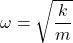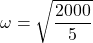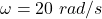Relation between velocity, Amplitude is given by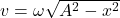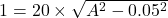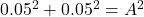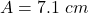Amplitude of the motion is equal to 7.1 cm.